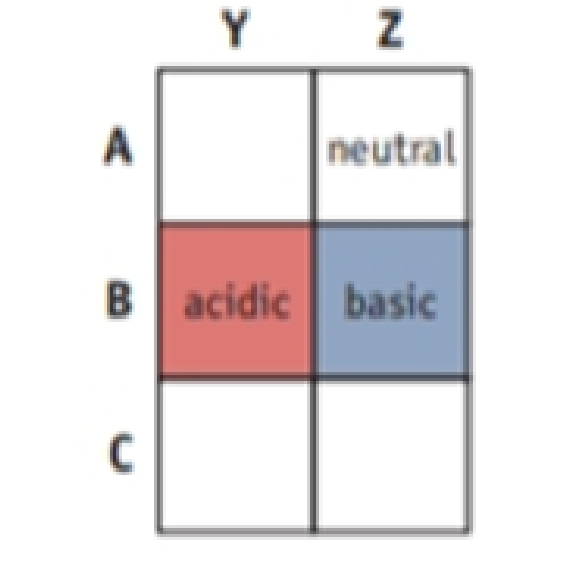Chapter 16, Problem 113IL

Chapter
Section
Textbook Problem

You have three solutions labeled A, B, and C. You know only that each contains a different cation—Na+, NH4+, or H3O+. Each has an anion that does not contribute to the solution pH (e.g., Cl−). You also have two other solutions, Y and Z, each containing a different anion, Cl− or OH−, with a cation that does not influence solution pH (e.g., K+). If equal amounts of B and Y are mixed, the result is an acidic solution. Mixing A and Z gives a neutral solution, whereas B and Z give a basic solution. Identify the five unknown solutions. (Adapted from D. H. Barouch: Voyages in Conceptual Chemistry, Boston, Jones and Bartlett, 1997.)Interpretation Introduction

Interpretation:

Among three solutions A, B, and C each contain a different cation and the other two solutions Y and Z each containing a different anion. Five unknown solutions are to be stated.

Concept introduction:

Metal cations have two characteristics that allow them to act as a Lewis acid:

(a) Their positive charge that attracts electrons.

(b) They have at least one empty orbital that can accommodate an electron pair.

Anions can act as a Lewis base because they have a lone pair of the electron that can donate to an empty orbital of Lewis acid and anion can also act as a bronsted base because they can accept a proton from an acid to form the conjugate acid of the ion.

A conjugate acid-base pair contains two compounds that differ only by a hydrogen ion and a charge of +1.

The stronger the acid, the weaker its conjugate base and vice-verca.

Explanation

Three solutions A, B, and C each containing different cations, Na+,NH4+ and H+ are

Lewis acid and two another solution Y and Z each containing different anions Cl,OH are Lewis bases.

A neutralization reaction can be defined as the reaction of an acid and base to produce salt and water. When a solution is neutralized it means that salt are formed from an equal amount of an acid and base. Depending on the acid-base properties of its ions, a salt can dissolve in water to produce a neutral solution, a basic solution, or an acidic solution.

There are three types of neutralization reaction as follows:

Strong Acid+StrongBaseSALT+H2O     pH=7(neutral)Strong Acid+WeakBaseSALT+H2O       pH<7(acidic)Weak Acid+StrongBaseSALT+H2O       pH>7(basic)

The Na+ cation is a Lewis acid, so it can combine with both Cl and OH ion. When a salt NaCl dissolves in water, it gives HCl and NaOH the reaction can be written as:

Na+(aq)+Cl(aq)+H2O(l)Na+(aq)+Cl(

Still sussing out bartleby?

Check out a sample textbook solution.

See a sample solution

The Solution to Your Study Problems

Bartleby provides explanations to thousands of textbook problems written by our experts, many with advanced degrees!

Get Started